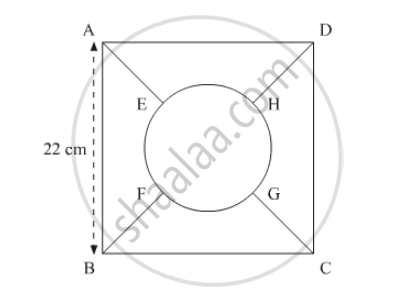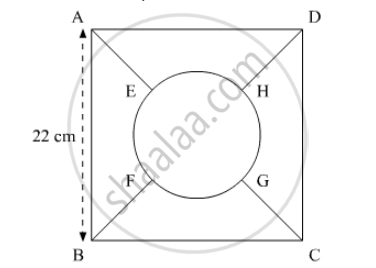Advertisement Remove all ads

# In the Following Figure, the Square Abcd is Divided into Five Equal Parts, All Having Same Area. the Central Part is Circular and the Lines Ae, Gc, Bf and Hd Lie Along the Diagonals Ac and Bd of - Mathematics

Sum

In the following figure, the square ABCD is divided into five equal parts, all having same area. The central part is circular and the lines AEGCBF and HD lie along the diagonals AC and BD of the square. If AB = 22 cm, find:

the perimeter of the part ABEF.Advertisement Remove all ads

#### Solution

We have a square ABCD.We have to find the perimeter of ABEF. Let O be the centre of the circular region.

Use Pythagoras theorem to get,

2(AE+r)^2=22^2

AE+r=15.56

AE=(15.56-5.56)cm

= 10c,

Similarly,

BF=10cm

Now length of arc EF,

= "perimeter of circular region"/4

= 34.88/4 cm

So, perimeter of ABFE,

=AB+BF+EF+AE

=(22+10+8.64+10)

= 50.64

Is there an error in this question or solution?
Advertisement Remove all ads

#### APPEARS IN

RD Sharma Class 10 Maths
Chapter 13 Areas Related to Circles
Exercise 13.4 | Q 17.2 | Page 58
Advertisement Remove all ads

#### Video TutorialsVIEW ALL 

Advertisement Remove all ads
Share
Notifications

View all notifications

Forgot password?
Course